• 最优的的四种查找算法

千次阅读 2020-04-20 22:52:28
顺序(线性)查找，二分查找/折半查找，插值查找，斐波那契查找

一，顺序(线性)查找

public void SeqSearch() {
int arr[] = {1, 9, 11, -1, 34, 89};// 没有顺序的数组
int value = 11,index = -1;
for (int i = 0; i < arr.length; i++) {
if (arr[i] == value)
index = i;
}
if (index == -1) {
System.out.println("can not find");
} else {
System.out.println("index = " + index);
}
}

二，二分查找/折半查找

public void BinarySearch(){
int arr[] = { 1, 2, 3, 4, 5, 6, 1,7, 8, 9, 10 };

int resIndex = binarySearch(arr, 0, arr.length - 1, 1000);
System.out.println("resIndex=" + resIndex);
}
public static List< Integer> binarySearch(int[] arr,int left,int right,int findVal) {
if (left > right) {
return -1;
}
int mid = (left + right) / 2;
int midVal = arr[mid];

if (findVal > midVal) { // 向 右递归
return binarySearch(arr, mid + 1, right, findVal);
} else if (findVal < midVal) { // 向左递归
return binarySearch(arr, left, mid - 1, findVal);
} else {
return mid;
}
}

三，插值查找

插值查找原理介绍:

1. 插值查找算法类似于二分查找，不同的是插值查找每次从自适应mid处开始查找。
2. 将折半查找中的求mid 索引的公式 , low 表示左边索引left, high表示右边索引right。key 就是前面的 findVal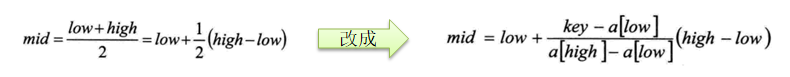3. int mid = low + (high - low) * (key - arr[low]) / (arr[high] - arr[low])插值索引
对应前面的代码公式：
int mid = left +(right -left) * (findVal-arr[left])/(arr[right]-arr[left])

插值查找注意事项：

1. 对于数据量较大，关键字分布比较均匀的查找表来说，采用插值查找, 速度较快.
2. 关键字分布不均匀的情况下，该方法不一定比折半查找要好
public void InsertValueSearch() {
int arr[] = {1, 8, 10, 89, 1000, 1000, 1234};
int index = insertValueSearch(arr, 0, arr.length - 1, 1000);
System.out.println("index = " + index);
}

public static int insertValueSearch(int[] arr, int left, int right, int findVal) {
//注意：findVal<arr与findVal>arr[arr.length-1] 必须需要,否则我们得到的 mid 可能越界
if (left > right || findVal < arr || findVal > arr[arr.length - 1]){
return -1;
}

int mid = left + (right - left) * (findVal - arr[left]) / (arr[right] - arr[left]);
int midVal = arr[mid];
if(findVal>midVal){
return insertValueSearch(arr,mid+1,right,findVal);
}else if(findVal<midVal){
return insertValueSearch(arr,0,mid-1,findVal);
}else{
return mid;
}
}

四，斐波那契查找

斐波那契(黄金分割法)查找基本介绍:

1. 黄金分割点是指把一条线段分割为两部分，使其中一部分与全长之比等于另一部分与这部分之比。取其前三位数字的近似值是0.618。由于按此比例设计的造型十分美丽，因此称为黄金分割，也称为中外比。这是一个神奇的数字，会带来意向不大的效果。
2. 斐波那契数列 {1, 1, 2, 3, 5, 8, 13, 21, 34, 55 } 斐波那契数列的两个相邻数 的比例，无限接近 黄金分割值0.618

斐波那契(黄金分割法)原理:

• 斐波那契查找原理与前两种相似，仅仅改变了中间结点（mid）的位置，mid不再是中间或插值得到，而是位于黄金分割点附近，即mid=low+Fib(k-1)-1（F代表斐波那契数列），如下图所示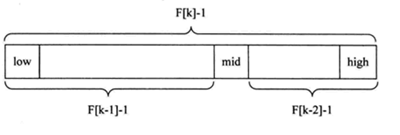• 对Fib(k-1)-1的理解：
1. 由斐波那契数列 Fib[k]=Fib[k-1]+Fib[k-2] 的性质，可以得到 (Fib[k]-1)=(Fib[k-1]-1)+(Fib[k-2]-1)+1 。该式说明：只要顺序表的长度Fib[k]-1，则可以将该表分成长度为Fib[k-1]-1Fib[k-2]-1的两段，即如上图所示。从而中间位置为mid=low+Fib(k-1)-1

2. 类似的，每一子段也可以用相同的方式分割。

3. 顺序表长度n不一定刚好等于Fib[k]-1，所以需要将原来的顺序表长度n增加至Fib[k]-1。这里的k值只要能使得Fib[k]-1恰好大于或等于n即可，由以下代码得到,顺序表长度增加后，新增的位置（从n+1到Fib[k]-1位置），都赋为n位置（最末尾的数）的值即可。

public void FibonacciSearch() {
int arr[] = {1, 8, 10, 89, 1000, 1000, 1234};
int key=1000;

int[] fib = new int;
fib = 1;
fib = 1;
for (int i = 2; i < 20; i++) {
fib[i] = fib[i - 1] + fib[i - 2];
}

int low = 0;
int high = arr.length - 1;
int k = 0; //表示斐波那契分割数值的下标
int mid = 0;
//获取到斐波那契分割数值的下标
while (high > fib[k] - 1) {
k++;
}
//因为 f[k] 值 可能大于 arr 的 长度,不足的部分会使用arr最后一位数填充
int[] temp = Arrays.copyOf(arr, fib[k]);
for (int i = high + 1; i < temp.length; i++) {
temp[i] = arr[high];
}

while(low<=high){
mid=low+fib[k-1]-1;
if(key < temp[mid]) {
high=mid-1;
k--;
}else if(key>temp[mid]){
low=mid+1;
k -= 2;
}else{ //找到
if(mid<=high){
System.out.println(mid);
}else{
System.out.println(high);
}
break;
}
}
}

展开全文java 算法 数据结构 索引 快速排序
• 主要介绍了Java实现的快速查找算法,结合具体实例形式分析了快速查找算法的原理与相关实现技巧,需要的朋友可以参考下
• 本文将对常见的查找算法进行总结。 2. 常见算法 2.1 顺序查找 基本思想： 该算法简单粗暴，从头（或是最后）开始遍历，找到要查的数据就停止遍历并返回结果，如果遍历完也没有找到就是查找不成功。 时间复杂度:O(n) ...

1. 简介

查找算是工作过程中运用最广泛的操作了，操作系统读取文件时需要查找，从数据库读取数据时需要查找…

本文将对常见的查找算法进行总结。

2. 常见算法

2.1 顺序查找

基本思想：

该算法简单粗暴，从头（或是最后）开始遍历，找到要查的数据就停止遍历并返回结果，如果遍历完也没有找到就是查找不成功。

时间复杂度:O(n)

2.2 有序表

2.2.1 二分查找

基本思想：

1. 将表中间位置记录的关键字与查找关键字比较，如果两者相等，则查找成功；
2. 否则利用中间位置记录将表分成前、后两个子表，
1. 如果中间位置记录的关键字大于查找关键字，则进一步查找前一子表，
2. 否则进一步查找后一子表。
3. 重复以上过程，直到找到满足条件的记录，使查找成功，或直到子表不存在为止，此时查找不成功

时间复杂度:O(log2n)

2.2.2 插值查找

对于数值变化幅度比较均匀的有序数组，要查的值在数组中的位置基本是可以确定的，例如[10,20,30,40,60,70,80,90,100,120,130,140]这个数组，30是在数组的前半部分，60应该是离30不远的位置，而130则是在数组的后半部分，120,140是在130附近。

二分查找法用在上面的数组中，对于位置的计算可能就存在优化的空间了，优化后的算法就叫插值查找法。

基本思想：基于二分查找算法，将查找点的选择改进为自适应选择，可以提高查找效率。插值查找是根据要查找的关键字key与查找表中最大最小记录的关键字比较后的查找方法，改进后的公式如下：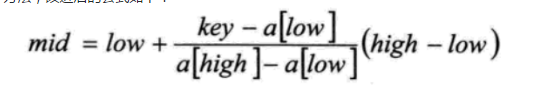时间复杂度：o(logn)

适用场景：对于表长较大而关键字分布比较均匀的查找表来说，效率高于二分查找法。

2.2.3 斐波那契查找

斐波那契数列如下所示：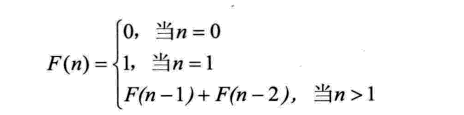斐波那契查找原理与前两种相似，仅仅改变了中间结点（mid）的位置，mid不再是中间或插值得到，而是位于黄金分割点附近，即mid=low+F(k-1)-1（F代表斐波那契数列），如下图所示：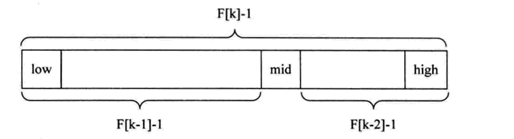基本思想：

由斐波那契数列 F[k]=F[k-1]+F[k-2]的性质，可以得到（F[k]-1）=（F[k-1]-1）+（F[k-2]-1）+1。该式说明：只要顺序表的长度为F[k]-1，则可以将该表分成长度为F[k-1]-1和F[k-2]-1的两段，即如上图所示。从而中间位置为mid=low+F(k-1)-1

但顺序表长度n不一定刚好等于F[k]-1，所以需要将原来的顺序表长度n增加至F[k]-1。这里的k值只要能使得F[k]-1恰好大于或等于n即可。

时间复杂度：O(log2n)。

理轮上，由于下述原因，该算法的平均性能会高于二分查找法：

1. 如果查找的记录在右侧，则查找的数据量会少一些
2. mid=low+F(k-1)-1. 是基于加减法的操作，数据量大的时候，效率上会高于除法。

2.3 无序数据

数据按照时间存储，值可能是无序的。

2.3.1 简单索引

基本思想：存储数据的时候，使用数据的key创建出有序的索引，索引中还保存指向原始数据的指针。查找的时候可以用有序表的算法找到索引，然后用索引中存储的数据指针找到原始数据。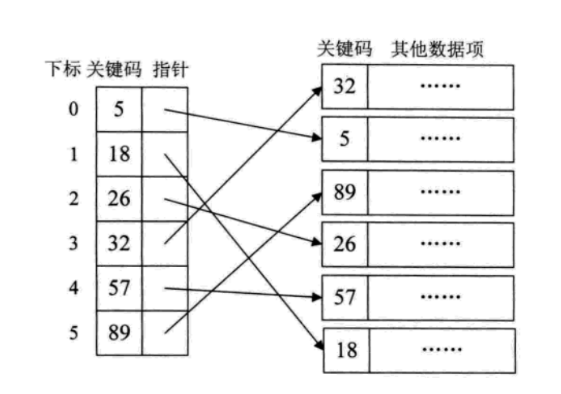2.3.2 分块索引

数据量大的时候，简单索引需要较多的内存空间去存储索引数据，此时可以考虑使用分块索引。

算法思想：将n个数据元素"按块有序"划分为m块（m ≤ n）。每一块中的结点不必有序，但块与块之间必须"按块有序"；即第1块中任一元素的关键字都必须小于第2块中任一元素的关键字；而第2块中任一元素又都必须小于第3块中的任一元素，……

索引结果如下图所示：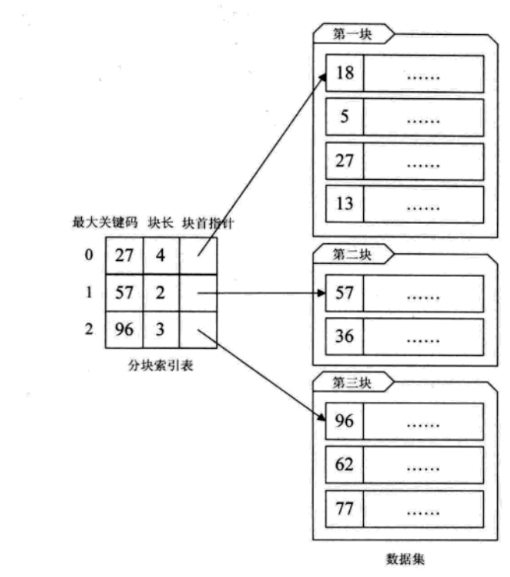查找流程：

1. 先选取各块中的最大关键字构成一个索引表；
2. 查找分两个部分：先对索引表进行二分查找或顺序查找，以确定待查记录在哪一块中；然后，在已确定的块中用顺序法进行查找。

2.3.3 倒排索引

解决文本搜索的必备技能，请参考：https://blog.csdn.net/eric_sunah/article/details/79404022 第五章节

2.3.4 哈希查找

请参考：https://blog.csdn.net/eric_sunah/article/details/85393235

2.3.5 B家族的树

曾转过一篇关于常见B系列树的介绍，包括：B树、B-树、B+树、B*树，请参考：https://blog.csdn.net/eric_sunah/article/details/86482113

2.3.6 红黑树查找

请参考：https://blog.csdn.net/eric_sunah/article/details/86482146

展开全文斐波那契查找 二分查找 哈希查找 倒排索引
• 六种查找算法效率比较

千次阅读 2014-04-03 15:37:26
由于查找一个数的过程，无论运用哪种算法对于电脑来说速度都是非常的，都在1ms之内，无法用计时函数测试出来。所以为了能够直观准确地表示出各个算法间的差异，此程序用了循环查找的方法，具体的思想是：先随机...
接着上次的
排序算法讨论，这次谈的是六种查找
算法，分别是：顺序查找、折半查找、二叉树查找、索引查找、开地址哈希查找方法、拉链法哈希查找方法。

由于查找一个数的过程，无论运用哪种算法对于电脑来说速度都是非常快的，都在1ms之内，无法用计时函数测试出来。所以为了能够直观准确地表示出各个算法间的差异，此程序用了循环查找的方法，具体的思想是：先随机生成3000个数作为查找的数据源，再随机生成3000（也可以少一点）个数作为被查找的数，让当前的查找算法运行一趟，这样就相当于运行了3000次。

这样还不具有一定的客观性，用flag标记出刚刚运行完查找后的结果，从数据源中找到目标的数标记为1，没找到的标记为0，并以此存为两个数组，最后我们就可以使用这两个数组再次分别进行循环查找，同时开始计时。如此一来就可以计算出各个算法在查找成功的情况下需要多少时间，反之在没查找到的情况下需多长时间了。代码如下：

#include <stdio.h>
#include <stdlib.h>
#include <time.h>
#include <windows.h>

#define ListSize 3000
#define TableSize ListSize*5/4
#define  NULLKEY  0

typedef int KeyType;

typedef struct
{
KeyType key;
}RecordType;

typedef struct node
{
KeyType key;
struct node *lchild,*rchild;
}BSTNode,*BSTree;

typedef struct
{
KeyType r[ListSize+1];
int Length;
}RecordList;

typedef struct  //索引表结点
{
int key;     //最大关键字域
}Index;

typedef struct   //顺序表结点
{
int key;     //关键字域
}Dexlist;

typedef struct Node{
int data;
struct Node *next;
}Node;

typedef RecordType HashTable[TableSize];
Dexlist dexlist[ListSize+1];
Index index;
RecordList list;
HashTable hashtable;
BSTree *bst;
Node **A;

int SeqSearch(RecordList l,KeyType k);
void InSort(RecordList *l,int length);
int BinSrch(RecordList l,KeyType k);
void InsertBST(BSTree *bst, KeyType x);
void CreateBST(BSTree *bst,RecordList list);
int SearchBST(BSTree bst,KeyType key);
int CreatIndex(Dexlist dl[],Index index[],RecordList list);
int IndexSearch(Dexlist r[],Index index[],int key);
int Hash(KeyType k);
void CreatHashTable(RecordList l,int SIZE);
int  HashSearch(HashTable ht, KeyType K,int SIZE);
void InitHash(Node **Q);
void InsertHash(Node **Q,int value);
int SearchHash(Node **Q,int value);

int CaseChoice(int choice,int x)
{
int i,k=0,flag=0;
bst=(BSTree *)malloc(sizeof(BSTree));
if(choice==1)
{
k=SeqSearch(list,x);
return k;
}
else if(choice==2)
{
InSort(&list,ListSize);
k=BinSrch(list,x);
return k;
}
else if(choice==3)
{
CreateBST(bst,list);
k=SearchBST(*bst,x);
return k;
}
else if(choice==4)
{
flag=CreatIndex(dexlist,index,list);
if(!flag)
{
printf("\n\n对不起！索引表创建失败\n");
exit(0);
}
k=IndexSearch(dexlist,index,x);
return k;
}
else if(choice==5)
{
CreatHashTable(list,TableSize);
k=HashSearch(hashtable,x,TableSize);
return k;
}
else if(choice==6)
{
A=(Node **)malloc(sizeof(Node*)*10); //申请一个指针型数组A[n]
InitHash(A);//初始化数组A
for(i=1;i<=ListSize;i++)
InsertHash(A,list.r[i]);//print_hash(A,n);
k=SearchHash(A,x);
return k;
}
else if(choice==7)
exit(0);
else
{
printf("\n    输入错误！\n");
exit(0);
}
}

void main()
{
int i,j,x,choice,k=0;
list.Length=ListSize;
srand((unsigned)time(NULL));
double runtime;
int T=0,F=0;
int success[ListSize],fail[ListSize];
long dwStart,dwEnd;
do{
printf("\n\n=========六种查找算法效率比较=========");//
printf("\n\n	1. 顺序查找");
printf("\n\n        2. 折半查找");
printf("\n\n        3. 二叉树查找");
printf("\n\n        4. 索引查找");
printf("\n\n        5. 开地址法哈希查找(α=0.8)");
printf("\n\n        6. 拉链法哈希查找");
printf("\n\n        7. 退出\n");
printf("\n======================================");
printf("\n    请输入您的选择 (1,2,3,4,5,6,7)：");
scanf("%d",&choice);
for(i=0;i<ListSize;i++)
{
j=1+(unsigned)(10000.0*rand()/(RAND_MAX+1.0));
list.r[i]=j;
}
bst=(BSTree *)malloc(sizeof(BSTree));
srand((unsigned)time(NULL));
for(i=0;i<ListSize;i++)
{
j=1+(unsigned)(10000.0*rand()/(RAND_MAX+1.0));
x=j;
k=CaseChoice(choice,x);
if(k==0)
{
fail[F]=x;
F++;
}
else
{
success[T]=x;
T++;
}
}

dwStart=GetTickCount();
for(i=0;i<F;i++)
k=CaseChoice(choice,fail[i]);
dwEnd=GetTickCount();
runtime=(double)(dwEnd-dwStart)/F;
printf("\n     查找了%d个数的数组，该种查找算法的平均查找失败时间是：%lf ms\n",ListSize,runtime);

dwStart=GetTickCount();
for(i=0;i<T;i++)
k=CaseChoice(choice,success[i]);
dwEnd=GetTickCount();
runtime=(double)(dwEnd-dwStart)/T;
printf("\n     查找了%d个数的数组，该种查找算法的平均查找成功时间是：%lf ms\n\n",ListSize,runtime);
}while(choice!=7);
}

int SeqSearch(RecordList l,KeyType k)
/*在顺序表l中顺序查找其关键字等于k的元素，若找到，则函数值为该元素在表中的位置，否则为0*/
{
int i;
l.r=k;
i=l.Length;
while(l.r[i]!=k)
i--;
return(i);
}   //顺序查找

void InSort(RecordList *l,int length)
/* 对记录数组r做直接插入排序，length为数组中待排序记录的数目*///直接插入排序
{
int i,j;
for(i=2;i<=length;i++)
{
l->r=l->r[i];//将待插入记录存放到监视哨r中
j=i-1;
while(l->r<l->r[j])/* 寻找插入位置 */
{
l->r[j+1]= l->r[j];
j=j-1;
}
l->r[j+1]=l->r; /*将待插入记录插入到已排序的序列中*/
}
}   //插入排序

int BinSrch(RecordList l,KeyType k)
/*在有序表l中折半查找其关键字等于k的元素，若找到，则函数值为该元素在表中的位置*/
{
int low,high,mid;
low=1;
high=l.Length;/*置区间初值*/
while(low<=high)
{
mid=(low+high)/2;
if(k==l.r[mid])
return(mid);/*找到待查元素*/
else
if(k<l.r[mid])
high=mid-1;/*未找到，则继续在前半区间进行查找*/
else
low=mid+1;/*继续在后半区间进行查找*/
}
return (0);
}   //折半查找

void InsertBST(BSTree *bst,KeyType x)
/*若在二叉排序树中不存在关键字等于key的元素，插入该元素*/
{
BSTree s;
if(*bst==NULL)/*递归结束条件*/
{
s=(BSTree)malloc(sizeof(BSTNode));/*申请新的结点s*/
s->key=x;
s->lchild=NULL;
s->rchild=NULL;
*bst=s;
}
else
if(x<(*bst)->key)
InsertBST(&((*bst)->lchild),x);/*将s插入左子树*/
else
if(x>(*bst)->key)
InsertBST(&((*bst)->rchild), x); /*将s插入右子树*/
}
void CreateBST(BSTree *bst,RecordList list)
{
int i;
*bst = NULL;
for(i=1;i<=ListSize;i++)
{
InsertBST(bst,list.r[i]);
}
}
int SearchBST(BSTree bst,KeyType key)/*返回1成功，返回0失败*/
{
BSTree q;
q=bst;
while(q)
{
if(q->key==key)
return 1;
if(q->key>key)
q=q->lchild;
else
q=q->rchild;
}
return 0;
}   //二叉查找

int CreatIndex(Dexlist dexlist[],Index index[],RecordList list)
{
int m,n,i;
int number;
for(i=1;i<=10;i++)
index[i-1].key=1000*i;
for(i=1;i<=10;i++)
number[i-1]=0;
for(m=1;m<=ListSize;m++)
{
i=0;
while((list.r[m]>index[i].key)&&(i<10))
i++;
number[i]++;
}
for(n=1;n<10;n++)

for(m=1;m<=ListSize;m++)
{
i=0;
while((list.r[m]>index[i].key)&&(i<10))
i++;
dexlist[n].key=list.r[m];
}
m--;
if(m==ListSize)
return 1;
else
return 0;
}
int IndexSearch(Dexlist r[],Index index[],int key)//key 为给定值，索引表为index..index[b]
{
int i,j;
i=0;
while((key>index[i].key)&&(i<10))
i++;
if(i>=10)
{
return 0;
}
if(i>0)
else
while((j<ListSize)&&(key!=r[j].key)&&(r[j].key<=index[i].key))
j++;
if(key==r[j].key)
return 1;
else
return 0;
}   //索引查找

int Hash(KeyType k)
{
int h;
h=k%(TableSize);
return h;
}
void CreatHashTable(RecordList l,int SIZE)
{
int i,j,hj,t;
int p;
for(i=0;i<SIZE;i++)
{
hashtable[i].key=NULLKEY;
}
for(i=1;i<=ListSize;i++)
{
fflush(stdin);
p=l.r[i];
j=Hash(p);
if(hashtable[j].key==NULLKEY)
hashtable[j].key=p;
else
{
for(t=1;t<SIZE;t++)
{
hj=(j+t)%(SIZE);
if(hashtable[hj].key==NULLKEY)
{
hashtable[hj].key=p;
t=SIZE;
}
}

}
}
}
int HashSearch(HashTable ht, KeyType K,int SIZE)
{
int h0;
int i;
int hi;
h0=Hash(K);
if(ht[h0].key==NULLKEY)
return 0;
else
if(ht[h0].key==K)
return 1;
else/* 用线性探测再散列解决冲突 */
{
for(i=0;i<SIZE-1;i++)
{
hi=(h0+i)%SIZE;
if(ht[hi].key==NULLKEY)
return 0;
else
if(ht[hi].key==K)
return 1;
}
return 0;
}
}   //开地址法哈希查找

void InitHash(Node **A)
{
int i;
for(i=0;i<15;i++)
{
A[i]=(Node *)malloc(sizeof(Node));
A[i]->data=0;
A[i]->next=NULL;
}
}
void InsertHash(Node **Q,int value)
{
int key;
Node *p,*q;
key=value%15;
if(Q[key]->next!=NULL)
{
p=Q[key]->next;
while(p->next!=NULL)
p=p->next;
q=(Node *)malloc(sizeof(Node));
q->data=value;
q->next=NULL;
p->next=q;
}
else
{
q=(Node *)malloc(sizeof(Node));
q->data=value;
q->next=NULL;
Q[key]->next=q;
}
}
int SearchHash(Node **Q,int value)
{
int key;
Node *p;
key=value%15;
if(Q[key]->next==NULL)
return 0;
else
{
p=Q[key]->next;
while(p!=NULL)
{
if(p->data==value)
return 1;
p=p->next;
}
return 0;
}
}     //拉链法哈希查找

可能大家已经发现了，为了实现上述思路，并把代码冗余量减少，我把各个函数的引用又单独放到了一个CaseChoice函数里，主函数就负责循环。虽然简洁了不少，但如此一来有个问题：就是计时的时候把查找之前的一些动作都计算进去了，比如折半查找中包含了插入排序、二叉树查找包含了树的建立、哈希查找包含了建立哈希表的过程等等，应该有比较好的解决方法，但实在不想深究，于是乎放弃了但不管怎么说，把运行后的数据结果处理成图表后明显感觉到了各个算法间效率的差异。顺便提一句，数据结构课程设计（基于C语言）终于随着本次作业的完成而结束了，痛苦啊，如果再给我一个题目的话我要疯了。。。

展开全文• 有序数组的最快的查找算法

千次阅读 2020-03-20 11:15:17
有一个有序的数组，有没有最快的方法查找到里面的一个元素是否存在？存在返回下标，不存在返回-1。 部分人回答用循环，多数人想到用数组的findIndex方法。 很少的人知道这个题是要用二分查找的。 这个题主要是想看...

最近面试问的比较多的一个题目是：
有一个有序的数组，有没有最快的方法查找到里面的一个元素是否存在？存在返回下标，不存在返回-1。

部分人回答用循环，多数人想到用数组的findIndex方法。
很少的人知道这个题是要用二分查找的。

这个题主要是想看一下应聘人员对数据结构和算法有没一下了解。

当然上面三种方式都可以实现这个需求，但是最优解还是二分查找法。

首先，循环和findIndex的是算法复杂度度是O（n）,而二分查找的算法复杂度是O （logn）。
比如数组里面放1-10000的数值，那么找到9999这个数的话，二者的时间是多少呢？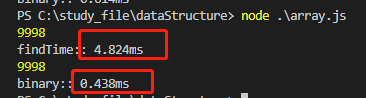这个数值是变动的，我们关注数量级。findIndex的方式是4ms多，二分的话是0.4ms,差了10倍左右。
如果是1000000个数值呢？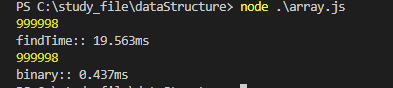可以看出二分查找的时间上优势。

测试代码：

let arr = [];
for (let i = 0; i < 1000000; i++) {
arr.push(i+1)
}

console.time('findTime:');
console.log(arr.findIndex(item=>item===999999))
console.timeEnd('findTime:');

console.time('binary:')
console.log(binarySearch(arr,999999))
console.timeEnd('binary:')

function binarySearch(arr, key) {
let start = 0;
let end = arr.length-1;
// let mid = Math.floor((start + end) / 2)
//     console.log(mid);
let mid ;
while (start <= end) {
mid = parseInt((start + end) / 2)
if (key < arr[mid]) {
end = mid-1;
} else if (key > arr[mid]) {
start = mid+1
}else if (key === arr[mid]) {
return mid//返回下标
}else{
return -1
}
}
}

展开全文哈希算法
• KMP 模式串匹配 指针 不回退 最快的字符串查找算法之一。 C++ builder6 调试通过。
• 七大查找算法详解

千次阅读 多人点赞 2019-08-12 11:32:31
本文详细介绍了七大查找算法，并且给出了相关的程序示例。查找算法
• 顺序查找算法

千次阅读 2021-05-09 16:30:17
顺序查找是按照序列原有顺序对数组进行遍历比较查询的基本查找算法。基本原理：对于任意一个序列以及一个给定的元素，将给定元素与序列中元素依次比较，直到找出与给定关键字相同的元素，或者将序列中的元素与其...算法 查找算法 顺序查找
• 六大查找算法（Python 语言实现）

千次阅读 多人点赞 2021-05-22 13:11:34
碰撞与溢出问题五、分块查找算法六、斐波那契查找算法七、六种查找算法的时间复杂度 一、顺序查找算法 顺序查找又称为线性查找，是简单的查找算法。这种算法就是按照数据的顺序一项一项逐个查找，所以不管数据...算法 python 数据结构
• 7种查找算法解析

万次阅读 多人点赞 2015-08-11 15:03:45
查找成功时的平均查找长度为：（假设每个数据元素的概率相等） ASL = 1/n(1+2+3+…+n) = (n+1)/2 ; 当查找不成功时，需要n+1次比较，时间复杂度为O(n); 所以， 顺序查找的时间复杂度为O(n ) 。 C++实现源码：...排序 查找
• 有许多查找算法可供选择，其中既包括直截了当的顺序搜索，也包括效率极高但应用受限的折半查找，还有那些将原集合用另一形式表示以方便查找的算法。最后一类算法对于现实应用具有特别重要的价值，因为它们对于大型...c语言 性能 算法
• 常见快速搜索算法图解

万次阅读 2020-01-29 09:49:05
搜索是在一个项目集合中找到一个特定项目的算法过程。搜索通常的答案是真的或假的，因为该项目是否存在。搜索的几常见方法：顺序查找、二分法查找、二叉树查找、哈希查找哈希表 二叉树 算法 数据结构 python
• 『算法』哨兵查找算法

千次阅读 2019-07-30 08:56:35哨兵查找算法
• C++实现常用查找算法

千次阅读 2019-08-11 13:20:53
在日常编程和面试中，查找算法和排序算法需要非常熟练。本文用C++语言的语法来写常用的查找算法：顺序查找，二分查找， 一、顺序查找 1.1基本思想（有序无序皆可以） 1 从表中的第一个元素开始，依次与关键字比较。 ...
• c语言的七大查找算法，非常值得学习

万次阅读 多人点赞 2018-04-18 20:48:36
今天带着大家学习下，C语言七大查找算法!!!!!!!!!!这里我们首先看下算法的概念： 算法（Algorithm）是指解题方案的准确而完整的描述，是一系列解决问题的清晰指令，算法代表着用系统的方法描述解决问题的策略机制。...嵌入式 C语言 算法
• 【转】七大查找算法总结

千次阅读 2019-06-23 23:23:22
思路：也称为折半查找，属于有序查找算法。用给定值k先与中间结点的关键字比较，中间结点把线形表分成两个子表，若相等则查找成功；若不相等，再根据k与该中间结点关键字的比较结果确定下一步查找哪个子表，...查找
• 常见查找算法

万次阅读 2018-05-28 10:38:32
一、顺序查找二、折半查找(二分查找)基本思路: 选定这批数中居中位置的一个数 与所查数进行比较, 看是否为所找之数, 若不是,利用数据的有序性,可以决定所找的数是在选定前还是在之后, 从而很快可以将查找范围缩小...折半查找 二叉排序树
• 本文简单概括性的介绍了常见的七种查找算法，说是七，其实二分查找、插值查找以及斐波那契查找都可以归为一类——插值查找。插值查找和斐波那契查找是在二分查找的基础上的优化查找算法。树表查找和哈希查找会在...
• 终于找了个时间，把三静态查找算法简单总结了一下，与大家分享讨论。  完整源代码下载地址顺序查找简介  顺序查找是在一个已知无(或有序）序队列中找出与给定关键字相同的数的具体位置。原理是让关键字与队列...查找算法 静态查找
• 作者：runzhiwang，腾讯 TEG 后台开发工程师本文介绍一跳点搜索算法 JPS 以及其四个优化算法，其寻路速度最快可是 A*算法的 273 倍。文中的 JPS-Bit 和 JP...算法 java python webgl
• 查找是在大量的信息中寻找一个特定的信息元素，在计算机应用中，查找是常用的基本运算，例如编译...本文简单概括性的介绍了常见的七种查找算法，说是七，其实二分查找、插值查找以及斐波那契查找都可以归
• 数据结构–七大查找算法总结

万次阅读 多人点赞 2018-08-06 17:13:43
转 数据结构–七大查找算法总结 2017年08月15日 21:06:17 阅读数：10610 ...查找算法 数据结构
• C++常用查找算法总结（一）

万次阅读 多人点赞 2018-07-29 21:33:50
容易理解的查找算法，顺序查找法  说明：顺序查找适合于存储结构为顺序存储或链接存储的线性表。 　基本思想：顺序查找也称为线形查找，属于无序查找算法。从数据结构线形表的一端开始，顺序扫描，依次将扫描...算法
• JAVA-快速查找算法

千次阅读 2015-12-08 14:01:46
快速查找算法，可以根据想要找的是第几个大的数，每次循环都能固定下来一个数在数组完整排完序之后的位置，每次循环都能定一个数的位置，如果当前固定的数的位置和用户要找的第几个数匹配，则就直接返回。...算法
• @C语言实现各大查找算法（一） 直 奔 主 题 ！！！ 今天用c语言实现数据结构中的查找算法，我们先实现顺序查找、折半查找（二分查找）、插值查找算法，后续我们会实现其他的查找算法。（主要是咱也还不会啊…） 先...C语言 二分查找 插值查找 查找算法
• 有序查找的三种算法

千次阅读 2015-03-27 21:50:46
#include int Binary_Search(int *a,int n, int key) { int low,high,mid; low = 0; high = n; while(low) ... mid = low +(high-low)*(key-a[low])/(a[high] - a[low]);//插值查找 //mid = (low+high)/2插值查找 斐波那契查找 折半查找 数据 search
• 数据结构-查找算法总结

千次阅读 2017-09-07 18:45:18数据结构 查找算法...